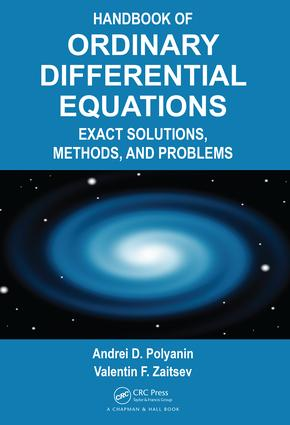# Handbook of Ordinary Differential Equations

## Exact Solutions, Methods, and Problems, 3rd Edition

Chapman and Hall/CRC

1,496 pages | 72 B/W Illus.

##### Purchasing Options:\$ = USD
Hardback: 9781466569379
pub: 2017-11-03
SAVE ~\$62.00
\$310.00
\$248.00
x
eBook (VitalSource) : 9781315117638
pub: 2017-11-15
from \$149.98

FREE Standard Shipping!

### Description

The Handbook of Ordinary Differential Equations: Exact Solutions, Methods, and Problems, is an exceptional and complete reference for scientists and engineers as it contains over 7,000 ordinary differential equations with solutions. This book contains more equations and methods used in the field than any other book currently available.

Included in the handbook are exact, asymptotic, approximate analytical, numerical symbolic and qualitative methods that are used for solving and analyzing linear and nonlinear equations. The authors also present formulas for effective construction of solutions and many different equations arising in various applications like heat transfer, elasticity, hydrodynamics and more.

This extensive handbook is the perfect resource for engineers and scientists searching for an exhaustive reservoir of information on ordinary differential equations.

EXACT SOLUTIONS OF ORDINARY DIFFERENTIAL EQUATIONS: First-Order Differential Equations. Second-Order Differential Equations. Third-Order Differential Equations. Fourth-Order Differential Equations. Higher-Order Differential Equations. Systems of Ordinary Differential Equations. SOLVING METHODS FOR ORDINARY DIFFERENTIAL EQUATIONS: First-Order Differential Equations. Second-Order Linear Differential Equations. Second-Order Nonlinear Differential Equations. Linear Equations of Arbitrary Order. Nonlinear Equations of Arbitrary Order. Analytic Ordinary Differential Equations and Their Local Classification. Lie Group and Discrete Group Methods. Linear Systems of Ordinary Differential Equations. Nonlinear Systems of Ordinary Differential Equations. Integrability of Polynomial Differential Systems. Hamiltonian Systems, Periodic, and Homoclinic Solutions by Variational Methods. Bifurcation Theory of Limit Cycle of Planar Systems. Successive Approximation Method for Nonlinear Boundary Value Problems. Application of Integral Equations for the Investigation of Differential Equations. Exact Methods for Construction of Particular Solutions for Nonlinear Equations. SYMBOLIC AND NUMERICAL SOLUTIONS OF ORDINARY DIFFERENTIAL EQUATIONS WITH MAPLE, MATHEMATICA, AND MATLAB: Ordinary Differential Equations with Maple. Ordinary Differential Equations with Mathematica. Ordinary Differential Equations with MATLAB. Supplements. References. Index.

### About the Authors

Andrei Polyanin received his Ph.D. from the Institute for Problems in Mechanics for the Russian Academy of Science where he currently teaches mathematics as a professor. His main research interest is with differential equaitons, fluid mechanics and applied and engineering mathematics.

Valentin F. Zaitsev is a professor at the Russian State Pedagogical University in St. Petersburg and the member of several mathematical journals as part of their editorial boards.

### Subject Categories

##### BISAC Subject Codes/Headings:
MAT000000
MATHEMATICS / General
MAT003000
MATHEMATICS / Applied
MAT004000
MATHEMATICS / Arithmetic
MAT007000
MATHEMATICS / Differential Equations